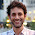## Tuesday, October 31, 2017

### The k=1 case is FUN, the k=2 case is fun, the k\ge 3 case is... you decide.

(All of the math in this post is in here.)

The following problem can be given as a FUN recreational problem to HS students or even younger: (I am sure that many of you already know it but my point is how to present it to HS students and perhaps even younger.)

Alice will say all  but ONE of the elements of {1,...,1010}in some order.

Bob listens with the goal of figuring out the number. Bob cannot possibly store 1010 numbers in his head. Help Bob out by giving him an algorithm which will not make his head explode.

This is an easy and fun puzzle. The answer is in the writeup  I point to above.

The following variant is a bit harder but a bright HS student could get it: Same problem except that Alice leaves out TWO numbers.

The following variant is prob more appropriate for a HS math competition than for a FUN gathering of HS students: Same problem except that Alice leaves out THREE numbers.

The following variant may be easier because its harder: Alice leaves out k numbers, k a constant. Might be easier then the k=3 case since the solver knows to NOT use properties of 3.

I find it interesting that the k=1, k=2, and k≥ 3 cases are on different levels of hardness.  I would like a more HS answer to the k≥ 3 case.

1.Here's a family of problems with different hardnesses for k=1,2,3,4,5: Given a planar graph, is it k-colorable? k=1: Is there an edge? k=2: Greedy algorithm. k=3: NP-hard. k=4: trivially yes; proof is somewhat long. k=5: proof is maybe two pages for a college student. (Is there an even easier proof that all planar graphs are 6-colorable?)

1.I think for k=6 there is an easier proof. It should follow from observation that every planar graph has a vertex of degree 5 (or less) via an easy induction.

2.Here's a family of problems with different hardnesses for k=1,2,3,4,5: Is a given planar graph k-colorable?

k=1: Is there an edge?
k=2: Greedy algorithm
k=3: NP-hard
k=4: Trivially yes; proof is somewhat long and tedious.
k=5: Proof is maybe two pages for a college student.
k>=6: Even easier?

3."Might be easier then the k=3 case since the solver knows to NOT use properties of 3."

Where you write "then" I believe you mean "than". Also, you can avoid splitting the infinitive with "knows NOT to use" rather than "knows to NOT use".

4.Two remarks:

1. One may argue that the case k=1 is the decisive one as the others are just technical embellishments using the same basic idea but more machinery (which you may not be familiar with).

2. A variant of the puzzle would be that Bob listens to a sequence of numbers and has to determine if they are all contiguous or contain holes. Same solution (remember the sum but just also remember min and max). What about naming all holes?

5.More variants:
(1) Count the number of holes, or in the original setting the missing numbers from the interval [0,n]
(2) Allow duplication on the side of Alice, i.e. she may repeat some of the numbers.

6.Suppose to be interested in a particular, informally stated decision problem, and to have formalised it by introducing an
alphabet Σ and an encoding scheme, mapping instances of the problem into strings from Σ
?
. As we have seen multiple times
during the course, the image of this encoding scheme gives raise to a language L, which is the formal representation of the
problem. Assume that the used encoding scheme possesses all the desirable properties— it is injective, and given x ∈ Σ
?
one can compute whether x is the code of some instance of the problem, and tell which one.
Suppose then that we manage to demonstrate that L is not decidable, and so we conclude that the problem is not solvable.
Would it be possible, by using a different encoding scheme (and possibly a different alphabet) to make the problem solvable?